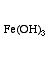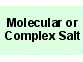Fe(OH)3
Ferric hydroxide
Iron(III) hydroxideFe 1 H 3 O 3

Real, long lived, electronically neutral reagent chemical.

Gram formula weight (molecular mass) = 106.86
Melting point = 500 °C decomp.
Density = 3.9 g/cm3
Purity = 99 %
Solubility in water = 2.070e-9 g/100mL
Solubility in organics = insoluble

 Wikipedia Page:Iron(III) hydroxide
is defined with respect to the entities below:
The entities below are defined with respect to:
Iron(III) hydroxide
 Molecular ionic material (generic)Type 23 Lewis acid/base complex (generic)chemical compound molecule metal molecular science reaction mechanism ionic material acid base geometry reactivity synthesis science knowledge chemistry Lewis